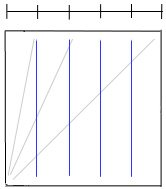# Divide Paper into Fifths: Method 3This method of dividing a square sheet of paper into equal fifths was first demonstrated by S Adams in this video; she used R Lang’s ReferenceFinder, to find the crease lines. This method of folding a sheet into fifths is probably the easiest; however, it leaves 3 crease marks.#### Divide Paper into Fifths: Method 3

• Fold and unfold a square sheet of paper in half along the diagonal.

• Looking at the top-left portion of the paper, fold in half again bisecting the angle at the bottom left.

• Repeat: fold and unfold the left edge of the paper to meet the second crease line thereby bisecting the lower-left angle one more time.

• The location where the third crease meets the top edge of the paper marks the 1/5 and 4/5 divisions. Make a vertical valley fold (and unfold) here.

• Fold and unfold the section that is 4/5-wide in half to get 1/5 + 2/5 + 2/5 divisions.

• Fold and unfold the 2/5-wide sections in half to achieve equal 1/5 divisions.